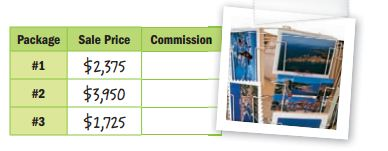Homework Explained - Math Practice 101Dear guest, you are not a registered member. As a guest, you only have read-only access to our books, tests and other practice materials.

As a registered member you can:

Registration is free and doesn't require any type of payment information. Click here to Register.
Go to page:
Chapter 2: Percents; Lesson 1: Percent of Number

• Question 12

In addition to her salary, Ms. Lopez earns a 3% commission, or fee paid based on a percent of her sales, on every vacation package that she sells. One day, she sold the three vacation packages shown. Fill in the table for each packages’ commission. What was her total commission?•  $Copy and Solve For Exercises 13–21, find each number. Round to the nearest hundredth. Show your work on a separate piece of paper. •  $$\frac{4}{5} \text{% of }500=$$ •  $$5\frac{1}{2} \text{% of }60=$$ •  $$20\frac{1}{4} \text{% of }3=$$ •  1,000 % of 99= •  520% of 100= •  0.15% of 250= •  200% of 79= •  0.3% of 80= •  0.28% of 50= ### H.O.T.Problems Higher Order Thinking • Question 22 Persevere with Problems Suppose you add 10% of a number to the number, and then you subtract 10% of the total. Is the result greater than, less than, or equal to the original number? Explain your reasoning. • Question 23 Reason Inductively When is it easiest to find the percent of a number using a fraction? using a decimal? • Question 24 Reason Inductively If you found the percent of a number and the product is greater than the number, what do you know about the percent? Explain. • Question 25 Marcos earned$300 mowing lawns this month. Of his earnings, he plans to spend 18% repairing lawn equipment, put 20% in his savings, and use 35% for camp fees. He will spend the rest. How much will Marcos have left to spend?• $27.00 •$55.00
• $81.00 •$100.00

Yes, email page to my online tutor. (if you didn't add a tutor yet, you can add one here)